Rectifiable PolyhexesPolyhexes cannot be rectifiable in the sense that they can form a larger copy of the basic form (a hexagon) but we can consider figures which approximate to the shape of a hexagon. Such a figure (an example is shown in the diagram below) must have an area equal to 3n2-3n+1 and must be non-prime. The only possibilities for n up to 100 are shown in the tables below where the number at the top of the table is the size of the polyhex which might tile the figure.Polyhexes in Hexagons

The diagram below shows a heptahex forming side 13 and 16 hexagons.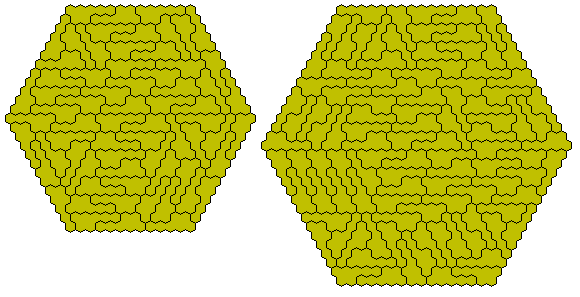This diagram shows how a hexagon can be extended by 7 using two basic blocks. There are two versions one for an odd sided hexagon and the other for an even sided one. This gives solutions for all hexagons with this piece except for sides 6 and 9.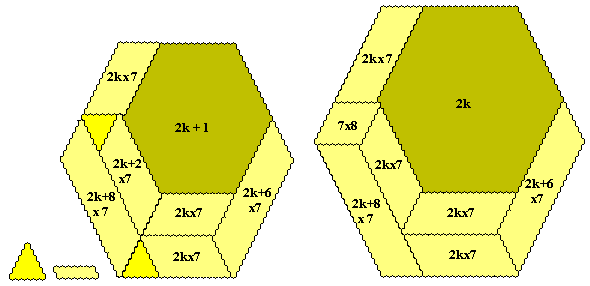Polyhexes in TrianglesOnly the one solution to the problem of tiling a hexagon with polyhexes is known but a slightly simpler problem does afford solutions. This is the problem of forming a 'triangle' with polyhexes. This was first suggested by Erich Friedman in his Problem of the Month (March 2003)

For a triangle to exist for an n-hex we must find a k for which ½k(k+1) is divisible by n. The tables below give the side of the triangle (first row), the total number of hexagons in the figure (second row) and the number of n-hexes needed to make the figure (bottom row).

In general if triangles of side n-1 and n exist then they can be combined to form triangles of sides 2n-1 and 2n as shown for the tetrahex below which also demonstrates how 2n-1 and 2n triangles can be used to extend a solution by 2n (some general methods of extension are shown below).More complicated extensions are shown below where a ring of triangles surrounds another.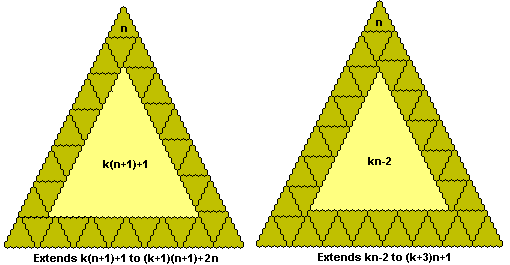There are two problems to be solved here. The main one is to determine which triangles can be formed. This will usually be an infinite set but in certain cases we find that some triangles can be combined to form others. Any triangle which can be made but not from smaller ones we shall refer to as prime and our secondary problem is to determine which triangles are prime. This is a much more difficult problem than with polyominoes as the combinations can be quite complex. The primes given are only those for which no combination of smaller triangles is known.

The above diagrams show that if n-1 and n sided triangles exist then any triangle of side (n-1)2 or greater can be extended by n thus showing that in these cases the number of primes will be finite. This is the situation in almost all known cases but the possibility of an infinite set of primes has not been excluded.

The dohex can form any triangle of side 4n-1 or 4n with the 3 and 4 being the only primes. The higher polyhexes are more complex and little is known in general.

Much of the data below have been supplied by Mike Reid.Trihexes:The trihex shown below forms triangles for sides 12k, 12k+2, 12k+9 and 12k+11. The construction at the right can be used to extend the triangles shown. This is the only trihex which can form a triangle.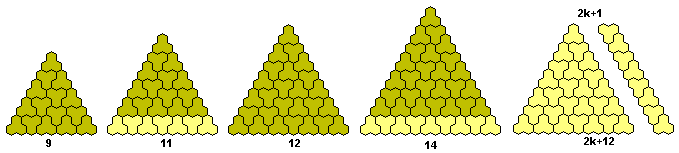Tetrahexes:There is only one tetrahex which can form triangles. This is possible for triangles of sides 8k-1 and 8k for all values of k. The constructions below can be extended to make any of these triangles thus showing that only the side 7 and side 8 triangles are prime.Pentahexes: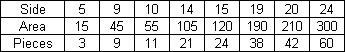Four pentahaxes are known to form triangles.5 is the only known solution.5k,5k+4 except for 4, 9, 10, 14 and 19 Primes are 5, 15, 20, 24, 25, 34, 54, 64, 84, 94, 114, 184, 214

As pointed out above the combinations of primes to form other triangles can be quite complex and the above list of primes probably contains some non-prime triangles. The following observations are by Mike Reid.Possible for all 5k (k = 1 and k > 2) and 5k+4 (k>1). The only primes are 5, 9, 14, 19, and 24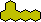Possible for all 5k (k = 1 and k > 2) and 5k+4 (k>1). The only primes are 5, 9, 14, 19 and 24.Hexahexes: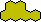Possible for all 12k (k>1) and 12k+3, 12k+8, 12k+11 (k>0).Possible for 24k, 24k+3(k>0),24k+20,24k+23Heptahexes: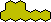7k, 7k-1 except for 7, 13, 14, 20. (35, 41 and 42 are open cases)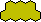7k and 7k-1 except for 7, 13, 14, 20. (35 is an open case)27k-1, 28k and possibly others

The construction at the right together with the side 28 triangle can be used to extend any triangle by 28.Octahexes:No solution is known for an octahex.

Pairs of Polyhexes in Triangles

As with the polyominoes and the polyiamonds some pairs of pieces which cannot themselves form a triangle can form triangles.